# Pentagons - polygons with 5 sides

By Martin McBride, 2022-10-10
Tags: pentagon 5 sided shape regular polygon irregular polygon
Categories: gcse geometryA pentagon is a flat shape with 5 straight sides.

## Regular and irregular pentagons

A regular pentagon is a 5-sided shape where every side is the same length and every corner has the same angle. All regular pentagons have the same shape, like this:An irregular pentagon is a 5 sides shape where not every side and angle are equal. There are many different irregular pentagon shapes, here is an example: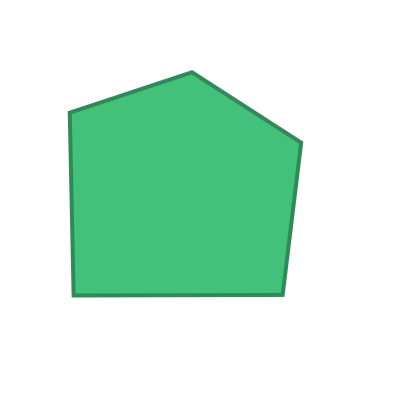## Name

The name pentagon is a combination of the words pente (Greek meaning five) and gonia (Greek meaning corner).

Pentagons are sometimes called 5-gons.

## Interior angles

The interior angles of a pentagon are shown here: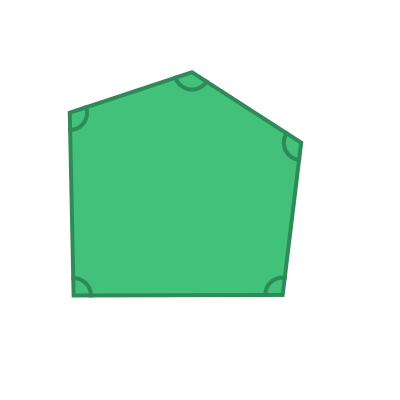The sum of these 5 angles is given by the formula:

sum of interior angles = (n - 2) x 180


Where n is the number of sides. In this case, the number of sides n is 5, so the sum of the interior angles is:

(5 - 2) x 180 = 540 degrees


For a regular pentagon, all the interior angles are equal:This means that the interior angle of a regular pentagon is:

540 / 5 =  108 degrees


## Exterior angles

The exterior angles of a pentagon are shown here: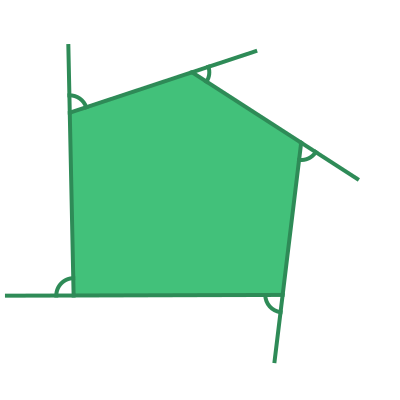The sum of the exterior angles of any polygon is 360 degrees.

For a regular pentagon, all the interior angles are equal: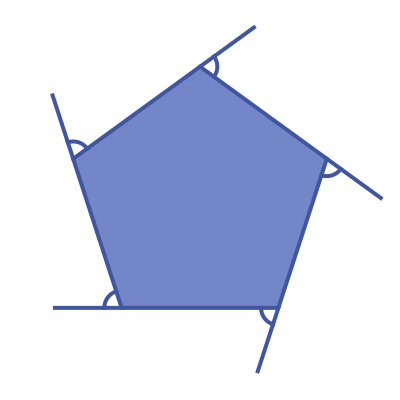This means that the exterior angle of a regular pentagon is:

360 / 5 =  72 degrees


## Symmetry of regular pentagons

A regular pentagon has 5 lines of symmetry. This means that it can be reflected over each of the 5 grey lines shown here: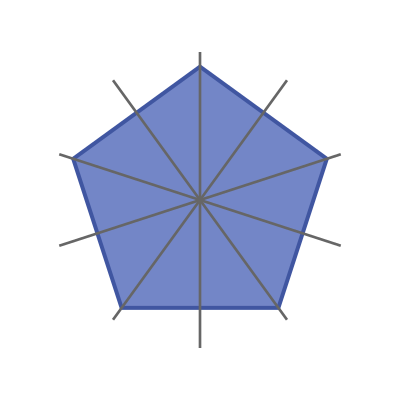A regular pentagon has rotational symmetry of order 5. This means that if it rotated about its centre by a 5th of a full turn, it will map onto itself: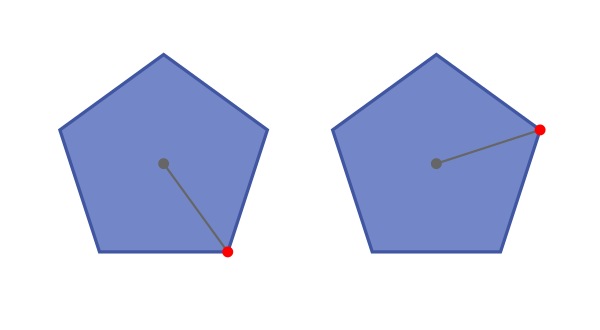## Real life examples

Pentagons appear quite often in nature. If you slice an apple in half horizontally and look at the cross section of its core, it forms a 5 pointed star whose points make a pentagon.